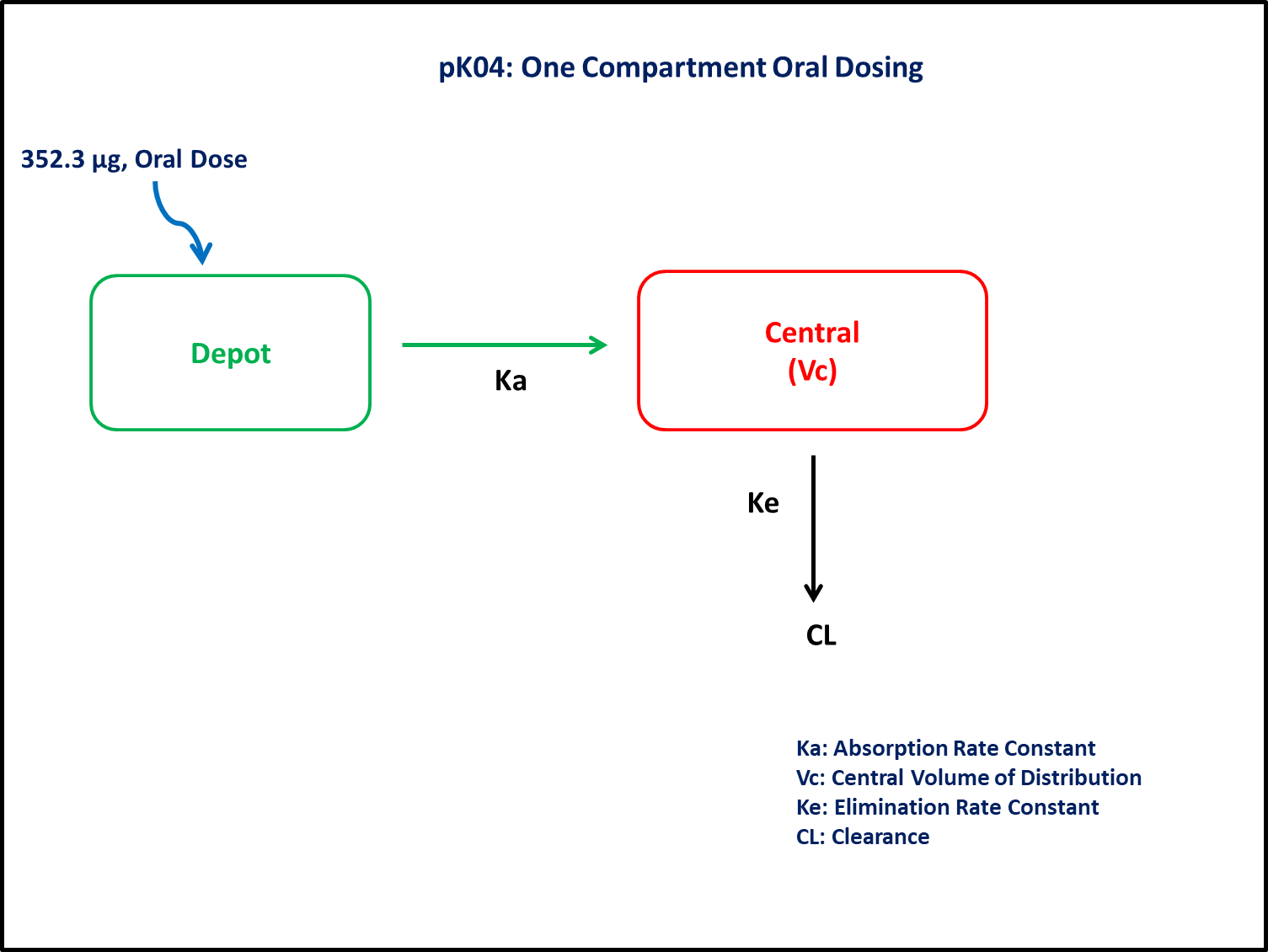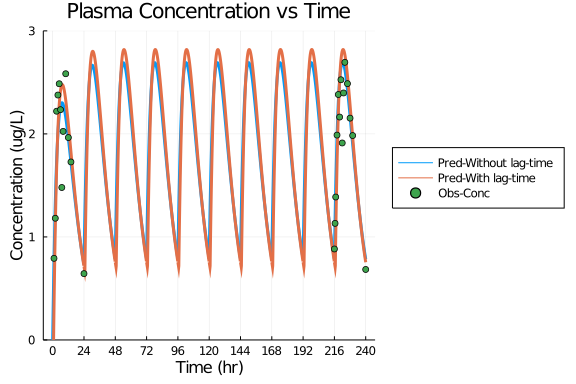# Exercise PK04 - One compartment oral dosing

### Background

• Structural model - One compartment linear elimination with first order absorption.

• Route of administration - Oral, Multiple dosing

• Dosage Regimen - 352.3 μg

• Number of Subjects - 1### Learing Outcome

This is a one compartment multiple oral dosing model. In the exercise pk04, four models are compared.

• Model 1 - One compartment model without lag-time, distinct parameters Ka and K

• Model 2 - One compartment model with lag time, distinct parameters Ka and K

• Model 3 - One compartment model without lag time, Ka = K = K¹

• Model 4 - One compartment model with lag time, Ka = K = K¹

### Objectives

In this tutorial, you will learn how to build one compartment model for multiple oral dosing and to simulate the model for a single subject.

### Libraries

call the "necessary" libraries to get start.

using Pumas
using Plots
using CSV
using StatsPlots
using Random


### Model 3 & 4

In this one compartment model, we administer multiple doses orally.

pk_04_3_4    = @model begin
@param begin
tvk¹     ∈ RealDomain(lower=0)
tvvc     ∈ RealDomain(lower=0)
tvlag    ∈ RealDomain(lower=0)
Ω        ∈ PDiagDomain(2)
σ²_prop  ∈ RealDomain(lower=0)
end

@random begin
η        ~ MvNormal(Ω)
end

@pre begin
K¹       = tvk¹ * exp(η)
Vc       = tvvc * exp(η)
lags     = (Depot = tvlag,)
end

@dynamics begin
Depot'   = -K¹*Depot
Central' =  K¹*Depot - K¹*Central
end

@derived begin
cp       = @. Central/Vc
dv       ~ @. Normal(cp, sqrt(cp^2*σ²_prop))
end
end

PumasModel
Parameters: tvk¹, tvvc, tvlag, Ω, σ²_prop
Random effects: η
Covariates:
Dynamical variables: Depot, Central
Derived: cp, dv
Observed: cp, dv


### Parameters

The parameters are as given below. tv represents the typical value for parameters.

• K¹ - Absorption and Elimination Rate Constant (hr⁻¹)

• Vc - Volume of Central Compartment(L)

• Ω - Between Subject Variability

• σ - Residual error

param3 = (tvk¹    = 0.14,
tvvc    = 56.3,
tvlag   = 0,
Ω       = Diagonal([0.0,0.0,0.0]),
σ²_prop = 0.015)

(tvk¹ = 0.14, tvvc = 56.3, tvlag = 0, Ω = [0.0 0.0 0.0; 0.0 0.0 0.0; 0.0 0.
0 0.0], σ²_prop = 0.015)

param4 = (tvk¹    = 0.15,
tvvc    = 52.3,
tvlag   = 0.68,
Ω       = Diagonal([0.0,0.0,0.0]),
σ²_prop = 0.01)

(tvk¹ = 0.15, tvvc = 52.3, tvlag = 0.68, Ω = [0.0 0.0 0.0; 0.0 0.0 0.0; 0.0
0.0 0.0], σ²_prop = 0.01)


### Dosage Regimen

Subject received 352.3 μg of oral dose once a day for 10 days.

ev1  = DosageRegimen(352.3, time=0, ii=24, addl=9, cmt=1)
sub3 = Subject(id=3, events=ev1)
sub4 = Subject(id=4, events=ev1)


### Simulation

Simulation the plasma concentration of the drug after multiple oral dosing

## Model 3 - without lag time
Random.seed!(123)
sim_sub3 = simobs(pk_04_3_4,sub3,param3,obstimes= 0:0.1:240)
df3      = DataFrame(sim_sub3)

## Model 4 - with lag time
Random.seed!(123)
sim_sub4 = simobs(pk_04_3_4,sub4,param4,obstimes= 0:0.1:240)
df4      = DataFrame(sim_sub4)


### Plots

df4_dv = filter(x -> x.time in [1,2,3,4,5,6,7,8,10,12,14,24,216,216.5,217,218,219,220,221,222,223,224,226,228,230,240], df3)

@df df3 plot(:time, :cp,
xlabel = "Time (hr)", ylabel = "Concentration (ug/L)", label = "Pred-Without lag-time",
title = "Plasma Concentration vs Time", linewidth = 3, legend=:outerright,
xticks = [0,24,48,72,96,120,144,168,192,216,240], ylims=(0,3.0))
@df df4 plot!(:time, :cp, label = "Pred-With lag-time", linewidth=3)
@df df4_dv scatter!(:time, :dv, label="Obs-Conc")